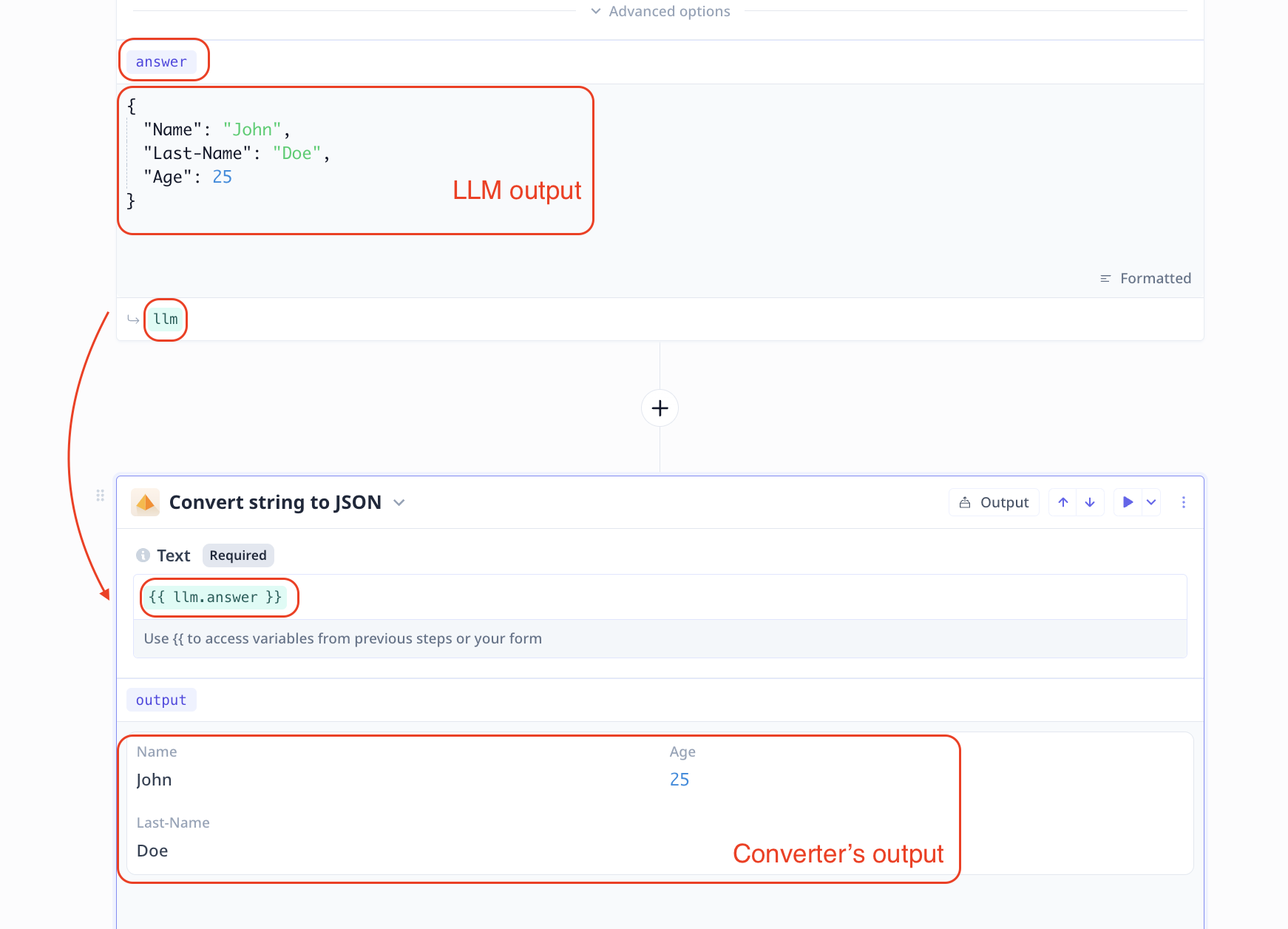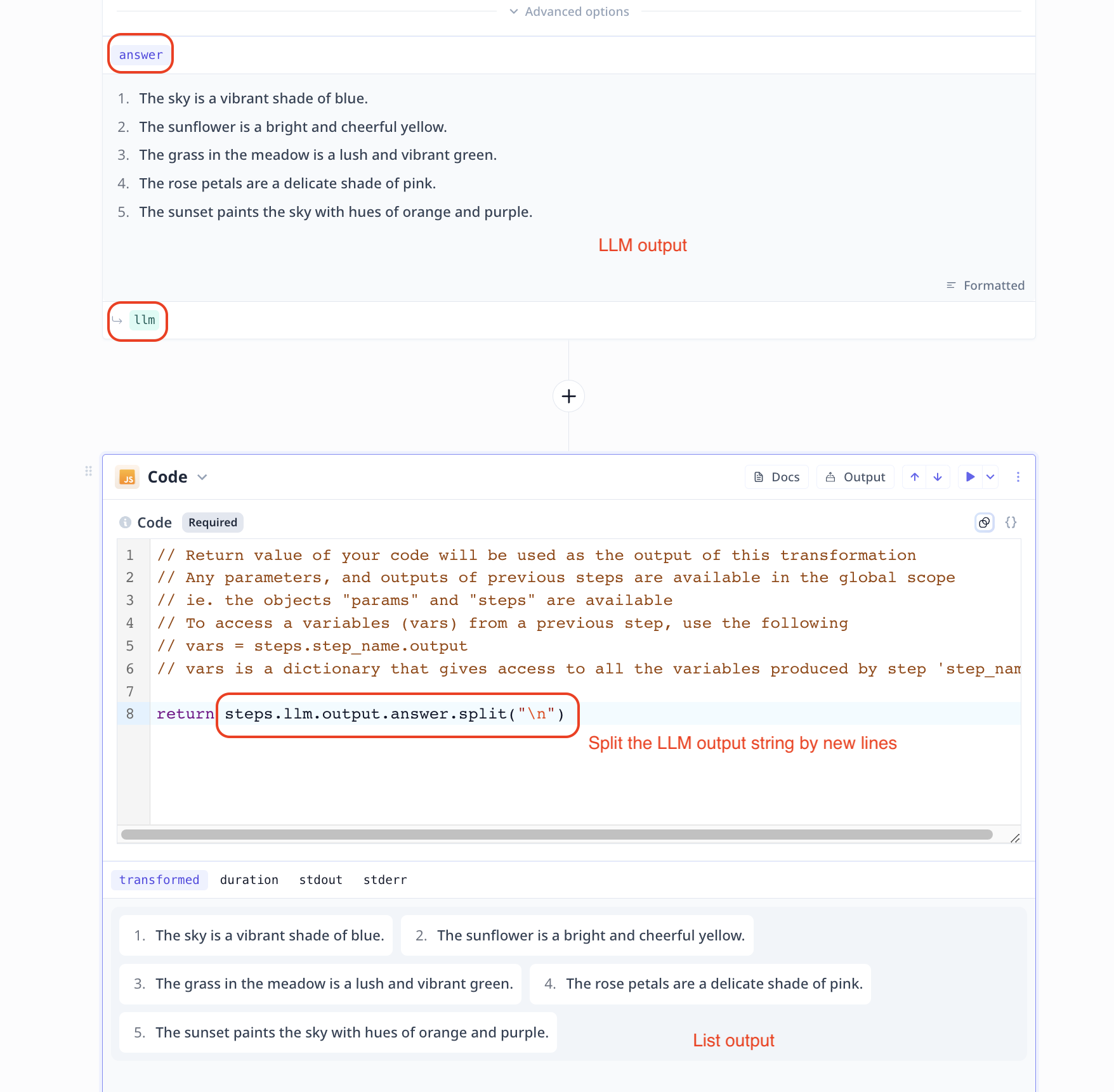Strings, the default output of LLMs, can be converted into other formats. For example, if you ask an LLM to produce a list of strings, you will need to convert the result into an array object to be able to analyze each element in a foreach loop individually.

On this page, we will note some of the common conversions. Please keep in mind that we use LLM output as a string example but the content applies to any string conversion.

### ​String to JSON

You can use a Convert string to json step to convert a JSON string to a JSON object. In the image below, we pass in the LLM step answer as input into the convertor step and the output is the corresponding JSON object.### ​String to list

Use a (JavaScript Code step or Python) Code step to handle the conversion.

All you need to do is to feed the string source value (e.g. LLM step answer) to your code step, apply the change (e.g. split(separator) in JavaScript) and return the value.

// if the separator is a comma like "a,b,c,d" -> "['a', 'b', 'c', 'd']"### ​String to Numbers or Booleans

Use a (JavaScript Code step or Python) Code step to handle the conversion.

All you need to do is to feed the string version of the input (e.g. LLM step answer) to your code step.

For numbers apply the parser (e.g. parseInt(string-value) in JavaScript) and return the results.

return parseInt(llm.answer);


For Booleans, you can simply use a conditional statement (if ... else ...) to return the corresponding Boolean value (True or False).

return llm.answer === 'true';